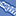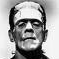# QlikView App Dev

Discussion Board for collaboration related to QlikView App Development.

Announcements
Join this live chat April 6, 10AM EST - QlikView to Qlik Sense REGISTER
cancel
Showing results for
Search instead for
Did you mean:Creator

## Help with number in text

Hi, could anyone help me get fix the number in the text?

below is my formula:

='2017 ('& ' \$(=\$(Max_Clos_2017))' & '+' & (12- '\$(=\$(Max_Clos_2017))')    & ')' &     '= R\$ '&

(Sum(Aggr(

(Sum(DISTINCT(if((left(Month,2)<= ('\$(=\$(Max_Clos_2017))'))and ('\$(=\$(Max_Clos_2017))')=Currency_Closing_Period ,Act_Rate))))

+

(Sum(DISTINCT(if((left(Month,2)> ('\$(=\$(Max_Clos_2017))')) and ('\$(=\$(Max_Clos_2017))')=Currency_Closing_Period ,Est_Rate))))

,Month)))/12

the result is: 2017 ( 5+7)= R\$ 3,215375. what change i should make so that the number would be 3,2153 only?

thanks

4 RepliesMaster II

Round(

Your Number Expression ,.0001)MVP

May be this

='2017 ('& ' \$(=\$(Max_Clos_2017))' & '+' & (12- '\$(=\$(Max_Clos_2017))')    & ')' &     '= R\$ '&

Floor((Sum(Aggr(

(Sum(DISTINCT(if((left(Month,2)<= ('\$(=\$(Max_Clos_2017))'))and ('\$(=\$(Max_Clos_2017))')=Currency_Closing_Period ,Act_Rate))))

+

(Sum(DISTINCT(if((left(Month,2)> ('\$(=\$(Max_Clos_2017))')) and ('\$(=\$(Max_Clos_2017))')=Currency_Closing_Period ,Est_Rate))))

,Month)))/12, 0.0001)Creator
Author

totally worked! thanksMaster

Hi Silvia,

Please remember to mark this question as Answered by Sunny.

Kind regards

Andrew